# Strong Interaction

Also found in: Dictionary, Thesaurus, Wikipedia.
Related to Strong Interaction: weak interaction, Residual strong interaction

## strong interaction

[′strȯŋ ‚in·tər′ak·shən]
(particle physics)
One of the fundamental interactions of elementary particles, primarily responsible for nuclear forces and other interactions among hadrons.
McGraw-Hill Dictionary of Scientific & Technical Terms, 6E, Copyright © 2003 by The McGraw-Hill Companies, Inc.
The following article is from The Great Soviet Encyclopedia (1979). It might be outdated or ideologically biased.

## Strong Interaction

one of the four basic interactions of nature. The others are the electromagnetic, gravitational, and weak interactions.

The particles that participate in strong interactions are known as hadrons. They contrast with particles that are not subject to strong interactions; the photon and the leptons, which include the electron, positron, muons, and neutrinos. Baryons, mesons, and a large number of unstable particles, or resonances, are classed as hadrons. The baryons include the nucleons—the neutron η and the proton p—and hyperons; the mesons include the pions, or π-mesons, and the kaons, or K-mesons.

One of the manifestations of strong interactions is the nuclear forces that bind nucleons in atomic nuclei. Strong interactions have a short range (~10-13 cm) and at such distances are considerably stronger than other types of interactions. Processes due to strong interactions occur in 10-23-10-24 sec. Strong interactions possess a high degree of symmetry. They are symmetric with respect to space inversion, charge conjugation, and time reversal. In addition, hadrons are characterized by internal symmetries that are specific to strong interactions: isotopic-spin invariance, symmetry with respect to a phase transformation (this symmetry leads to the existence of the special conserved quantum number called strangeness), and SU(3) symmetry.

Strong interactions, as forces of a new, previously unknown nature, were first detected in experiments by E. Rutherford in 1911, simultaneously with the discovery of the atomic nucleus. These forces account for the observed scattering of alpha particles at large angles as the particles pass through matter. The concept of strong interactions, however, was formulated later, principally in the 1930’s, in connection with the problem of the nuclear forces.

Short-range character. The most important feature of strong interactions is their short-range character. As noted above, strong interactions are manifested appreciably only at distances of the order of 10-13 cm between the interacting hadrons —that is, atomic dimensions are approximately 100,000 times greater than the range of strong interactions. At such distances, strong interactions are 100-1,000 times stronger than the electromagnetic forces acting between charged particles. As the distance increases, strong interactions diminish rapidly (approximately exponentially). At a distance that is a few times greater than their range, they are comparable to electromagnetic interactions; at still greater distances, strong interactions practically vanish. The short range of strong interactions explains why they were not experimentally detected until the 20th century, although they play an extremely important role in nature. By contrast, the weaker long-range electromagnetic and gravitational forces were detected and studied much earlier. (Because of their long-range character, the electromagnetic and gravitational forces exerted by a large number of particles are added to each other, and an interaction between macroscopic bodies thereby arises.)

To explain the short range of the nuclear forces, the Japanese physicist H. Yukawa in 1935 advanced the hypothesis that the strong interaction between nucléons (N) is a result of the nucleons’ exchange of some particle possessing mass. This exchange is carried out in a manner analogous to that for the electromagnetic interaction between charged particles, which is accomplished, according to quantum electrodynamics, through the exchange of photons, or particles of light (seeQUANTUM FIELD THEORY). It was proposed by Yukawa that there exists a specific interaction leading to the emission and absorption of an intermediate particle, which is the carrier of the nuclear forces. In other words, a new type of interaction, later named the strong interaction, was introduced. It should be noted that I. E. Tamm and D. D. Ivanenko independently first used the hypothesis of the exchange character of nuclear forces to explain the short range of such forces.

On the basis of the known experimental range of the nuclear forces, Yukawa estimated the mass of the particle carrying the strong interaction. This estimate was based on simple quantum-mechanical considerations. According to quantum mechanics, the relation between the time of observation Δt of a system and the indeterminacy in the system’s energy Δ£ is given by the uncertainty principle: ΔEΔt ~ , where is Planck’s constant. Therefore, if a free nucleon emits a particle of mass m (that is, if the energy of the system changes, according to the formula of the theory of relativity, by the amount ΔE = mc2, where c is the speed of light), then this process can occur only in the time Δt ~ /mc2. During this time, a particle moving with a speed approaching the maximum possible speed, namely, that of light c, can traverse a distance of the order of /mc. Consequently, for an interaction to occur between two particles through the exchange of a particle of mass m, the distance between the two particles must be of the order of, or less than, /mc— that is, the range of the forces carried by the particle of mass m must have the magnitude /mc. When the range is — 10-13 cm, the mass of the carrier of the nuclear forces should be ~300 me (where me is the mass of the electron), or approximately one-sixth the mass of a nucleon. Such a particle, the π-meson, was detected in 1947. The interaction was subsequently found to be much more complicated. It is carried not only by the charged π± - and neutral π0-mesons of masses 273me and 264me, respectively, but also by a large number of other mesons of greater mass, such as ρ, ω, Φ, and K. Moreover, the exchange of nucleons and antinucleons and of the excited states of such particles—baryon resonances—makes a certain contribution to strong interactions between, for example, mesons and nucleons. It follows from the uncertainty principle that the exchange of particles with masses greater than the mass of the pion occurs at distances less than 10-13 cm. In other words, such exchange determines the character of strong interactions at short distances. In principle, the contribution made to strong interactions by the exchange of specific particles can be determined from experimental study of various reactions involving hadrons, for example, such charge transfer reactions as π- + p → π0 + n and K- + p → K0 + n.

Relative magnitude of strong interactions. The magnitude of strong interactions can be characterized by comparing them with electromagnetic interactions, for the description of which an elaborate mathematical apparatus exists. Such a comparison makes possible an understanding of the difficulties encountered in the development of the theory of strong interactions. The interaction of a charged particle with an electromagnetic field, or photon field, is determined by the particle’s electric charge e, which also appears as the electromagnetic interaction constant. When charged particles interact, the probability of the emission of one photon is proportional, according to quantum electrodynamics, to the dimensionless quantity α = e2/ℏc ≈ 1/137, which is called the fine-structure constant. The probability of the emission of n photons in some process is proportional to αn—that is, is 1/137 of the probability of the emission of (n - 1) photons. (The emission of a large number of infrared photons of very small energy is an exception that requires special consideration.) Because α is small, the processes of the electromagnetic interaction can be investigated by means of perturbation theory. In this approach, we successively allow for the exchange of an increasingly large number of photons between the charged particles. Mathematically, such a theory is represented in the form of an infinite asymptotic power series in the small parameter α. Perturbation theory provides excellent agreement with experiment.

To characterize a strong interaction, such as the interaction of nucleons with a field of π-mesons, a constant g is introduced. Known as the strong coupling constant, g has the dimension of electric charge. As experiment shows, the dimensionless quantity g2/ℏc in strong interactions (which is analogous to the quantity α in electromagnetic interactions) is greater than unity: g2/ℏc ≈ 15. This fact means that the exchange of a large number of particles should play an important role in strong-interaction processes: when the energy of the colliding hadrons is sufficiently great, multiple processes with the production of a large number of secondary particles should predominate. Perturbation theory, which is so effective for electromagnetic interactions, therefore cannot be used for strong-interaction processes, and allowance must be made for the participation of a large number of particles in the interaction.

In certain branches of physics, such as solid-state physics, there are effective approximate methods of considering dynamic problems while allowing for many particles when the interaction between the particles is not small. Successful theoretical consideration of problems of this type is possible because the zero-order approximation for the state of the system is known, and states that are not strongly excited can be represented as a set of elementary excitations, or quasiparticles, whose interaction can be ignored in the zero-order approximation. For example, the thermal vibrations of the atoms of a solid can be represented as a set of vibrations of the crystal lattice as a whole: to these vibrations there correspond the quasiparticles known as phonons. The lack of a consistent theory of strong interactions may therefore be due to insufficient experimental data on the processes induced by such interactions. Further experimental and theoretical investigations may help find the zero-order approximation for describing the strong-interaction process.

Despite the lack of a consistent theory of strong interactions, a large number of relations between different strong-interaction processes have been established theoretically. The existence of relations of this type follows from the general principles of quantum field theory and from the existence of exact and approximate symmetries inherent in strong interactions. In addition, various semiphenomenological models of strong interactions are of great importance. These models permit a qualitative and, in many cases, a rather exact quantitative description of strong-interaction processes and make possible the prediction of new phenomena.

Strong interactions and the structure of hadrons. It follows from quantum-mechanical considerations analogous to those used in estimating the range of the nuclear forces that hadrons must be surrounded by a cloud of virtual pions and other virtual hadrons (seeVIRTUAL PARTICLES). These virtual particles are continually being emitted and absorbed. The radius of the pion cloud must be of the order of ℏ/ μ c, where μ is the mass of the pion, and the radii of the clouds produced by heavier hadrons must be inversely proportional to the masses of the hadrons. Because g2/c is large, the probability of the virtual emission of hadrons is great—that is, the clouds must have a considerable density and must play an important role in physical processes involving hadrons. In other words, it follows from the large value of the strong coupling constant that hadrons must have a complex internal structure and can be called elementary particles only conditionally. This conclusion holds even if we disregard the possibility that they consist of the more fundamental particles known as quarks.

Strong interactions and electromagnetic characteristics of hadrons. Strong interactions have a substantial effect on the electromagnetic characteristics of hadrons. The law of the conservation of electric charge requires that the charge of a hadron, including the total charge of the surrounding clouds, remain invariant, regardless of what virtual transformations occur in the clouds. Thus, strong interactions do not affect the electric charges of hadrons, which are integral multiples of the elementary electric charge e. The motion of the charges in the clouds, however, generates an electric current and consequently should lead to a change in the magnetic moments of the hadrons. This conclusion is in qualitative accord with measurements of the magnetic moments of nucleons. The magnetic moment of the proton is μp ≈ 2.79 μnucl where μnucl is the nuclear magneton, and the magnetic moment of the neutron is μn ≈ – 1.89 μnucl (the minus sign indicates that μn is directed opposite to the intrinsic angular momentum, or spin, of the neutron. If the proton and neutron did not have strong interactions, then, according to the Dirac equation, their magnetic moments would be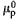= μnucl and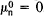, respectively. Therefore, if we assume that the anomalous magnetic moment of the neutron is created by a cloud of negatively charged mesons formed, for example, in the virtual transformations n → p + π- → n, then the anomalous moment of the proton should be generated through similar virtual transformations of the proton into positively charged mesons—for example, p → n + π+ → p. It can be shown that the intensity of such transitions is the same for the neutron and the proton. Thus, the anomalous magnetic moment of the proton should be equal in absolute value to the anomalous magnetic moment of the neutron and should be of opposite sign—that is, the sum of μp + μn should be close to μnucl This conclusion is in qualitative agreement with the experimentally measured values of the magnetic moments: μp + μn ≈ 0.9 μuncl. It may be noted that the quark model requires the ratio μnp to be equal to -2/3; this prediction is also well satisfied by the measured values of the magnetic moments.

Since a hadron is surrounded by a meson cloud, the hadron’s charge and magnetic moment should be distributed with a certain density throughout the region occupied by the cloud. In constant or slowly varying electromagnetic fields the dimensions of hadrons have practically no effect on the electromagnetic interactions in which the hadrons participate. The interactions in this case are determined entirely by the charges and magnetic moments of the hadrons. If, however, the dimensions of field nonuniformities, such as the de Broglie wavelength of electrons or photons interacting with a hadron, are less than the dimensions of the meson cloud, then the distribution of charge and magnetic moment within the hadron have a considerable effect on the nature of the interaction. Through investigation of the elastic scattering of electrons of energy greater than a few gigaelectron volts (GeV) by protons and deuterons, it is possible to determine experimentally the functions characterizing the spatial distribution of charge and magnetic moment inside nucléons. These functions are known as form factors. The results of experimental measurement of the form factors of the nucleons indicate that the densities of charge and magnetic moment are smoothly distributed throughout the region occupied by the cloud and decrease toward its periphery. Also, the charge and magnetic moment inside the proton are distributed in approximately the same way; this distribution is similar to the distribution of the magnetic moment of the neutron. At the same time, there is no experimental evidence for the existence within the nucleons of a distinct core, whose dimensions would exceed a few hundredths of the dimensions of the nucleon. Because of the loose structure of the cloud, the probability is extremely small that a large amount of momentum will be transferred to the cloud as a whole when electrons are elastically scattered by nucleons. The probability decreases rapidly with increasing transferred momentum.

If a large amount of momentum is transferred to the hadrons, then inelastic processes are much more probable. Here, a large number of secondary particles are knocked out of the cloud surrounding the hadron, and the electrons lose an appreciable part of their energy (such processes are said to be deep inelastic processes). In contrast to elastic scattering processes, the probability of the transfer of large momenta from electrons to hadrons is considerable. M. A. Markov was the first to suggest that deep inelastic processes exhibit such behavior. The experimentally measured structure functions characterizing the behavior of hadrons in deep inelastic processes depend only on the ratio of the square of the momentum transferred to the hadron cloud and the energy lost by the electron. Thus, the following similarity law holds: structure functions do not change if the transferred energy increases with transferred momentum. Theoretical support for this relationship follows from current algebra. With certain assumptions, such support is also obtained from the general principles of quantum field theory. A simple interpretation of the experimental data on deep inelastic scattering also follows from the parton model of R. Feynman. According to this model, a hadron behaves in deep inelastic processes as an aggregate of point particles, or partons, that are distributed in some way with respect to momentum. Quarks can be regarded as partons by assuming that the hadron contains, in addition to three quarks (as proposed in the first quark hypothesis), a cloud of quarks and antiquarks.

Because of the short-range character of the strong interaction, direct experimental study of the interaction is possible only in microparticle scattering processes. For scattering to occur, the impact parameter in this case must not exceed the range of the forces. It follows that the maximum relative angular momentum of the particles at which scattering can still occur is determined by the quantity ǀpǀR0, where p is the relative momentum of the particles and R0 is the range of the forces. In other words, waves with orbital momenta l ≤ ǀp\R0/ participate in the scattering process. The quantity k = ǀpǀ/ is called the wave number, the relation between it and the de Broglie wavelength ƛ = ℏ/ǀpǀ is given by the equation k = 1/ƛ.

At low energies, where kR0 < 1, scattering occurs in a state with orbital momentum l = 0 (in an S-wave) and is spherically symmetric—that is, occurs at any angle with equal probability. The range of energies E in which this condition is satisfied is restricted to values of E ≤ (10-15) million electron volts (MeV). In the indicated range the scattering process is completely described by two parameters: the scattering length and the effective range of the interaction. At higher energies, where kR0 ~ 1, phase shifts can be effectively used to describe the scattering process. The experimental determination of phase shifts in scattering processes provides important data on strong interactions. When the collision energy exceeds the secondary particle production threshold, inelastic reactions begin to predominate in strong-interaction processes. In the energy range where a small number of partial waves are involved in scattering, pronounced peaks are observed in the effective scattering cross section σ at energies corresponding to the formation of resonances. At energies exceeding a few GeV, the number of partial waves is great, and the contribution of resonances to the total cross section becomes negligible (Figure 1,A).

Inelastic processes at high energies. The conception of the hadron as a cloud of strongly interacting particles with a definite radius permits a qualitative understanding of strong interactions during the collision of high-energy hadrons. It is convenient to consider such collisions in a center-of-mass system of colliding particles, which is a coordinate system where the center of mass of the colliding particles is at rest—that is, where the particles move toward each other with momenta equal in magnitude and opposite in direction. Suppose that when two high-energy hadrons collide, they fly past each other in such a way that their clouds overlap. Because of the large value of the strong coupling constant, such collisions should be accompanied by the emission of a large number of secondary particles. The effective cross section of multiple processes consequently should be constant and equal to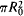, where R0 is the range of the strong interaction; in this graphic model the range is equal to the sum of the radii of the two colliding clouds. The kinematics of secondary particle production can also be easily represented on the basis of this simplified model. When the collision occurs, the two clouds are excited. As a result, the separation of the clouds is followed by the emission of secondary particles that, for the most part, move in the directions in which the two clouds separate (Figure 2). In addition, slower secondary particles may be emitted in various directions from the central region of the collision.

For a long time, cosmic rays were the only source of particlesFigure 1. Total effective cross sections σ for the scattering of π±-mesons, K±-mesons, protons (p), and antiprotons (ρ) by protons: (A) in the energy range up to 10 Gev, (B) at energies above 6 Gev; the different symbols indicate measurements in different accelerators

of energy greater than a few tens of GeV, and observed multiple processes were believed to conform approximately to this picture. In particular, measurements in a very broad energy range indicated the approximate constancy of the effective cross section of multiple processes. It was difficult to draw more accurate conclusions under the conditions of measurements with cosmic rays. Experiments conducted on high-energy accelerators at Serpukhov in the USSR, the European Center for Nuclear Research (CERN), and Batavia in the USA led to considerable refinements in the picture of multiple processes. It was established that the total effective cross sections for hadron interactions slowly decrease with increasing energy and become approximately constant at energies of a few tens of GeV. As the energy further increases, an increase is observed in the total scattering cross sections (see Figure 1,B); this increase was first observed in the scattering of K+-mesons by nucleons at the Serpukhov accelerator and is sometimes referred to as the Serpukhov effect. Experiment shows that an increase in the interaction cross sections σ is universal for hadrons and apparently approaches the maximum possible increase established on the basis of the general principles of present-day quantum theory: σ ~ ln2E, where E is the collision energy. This fact indicates that at high energies additional mechanisms of interaction show up that result in an increase in the range of strong interactions.

The study of high-energy multiple processes provides the key to understanding the dynamics of strong interactions. In this regard, the study of the special class of processes known as inclusive processes is of great importance. In such study, processes with the production of some specific secondary particles are singled out of a set of multiple events, and the angular and energy distributions for the particles are measured. These processes were first considered theoretically and proposed for study by Soviet physicists. A similarity law specific to inclusive processes has been discovered. Called scaling invariance, it requires the distribution of secondary particles by momenta to be the same for different collision energies if the momentum is measured in fractions of the maximum possible momentum for the given collision energy. Scaling invariance in hadron collisions, as in deep inelastic collisions of leptons with hadrons, can provide information on the characteristics of the interaction on the light cone—that is, when the interaction propagates with the maximum possible speed, the speed of light. Knowledge of these characteristics may be the decisive link for the construction of a theory of strong interactions.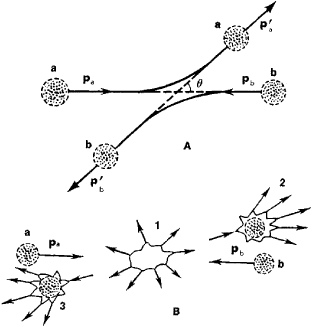Figure 2. Schematic of the collision of particles in their center-of-mass system: (A) elastic collision, (B) inelastic process; (1) central region of emission of secondary particles, (2) and (3) fragmentations of particles a and b

Elastic scattering of hadrons at high energy. Elastic processes are processes in which, as a result of an interaction, the colliding particles undergo a change only in their direction of motion—that is, the types of particles involved do not change, and there is no additional production of secondary particles. In a collision of high-energy hadrons where the hadrons approach to a distance less than the range of the strong interaction, the production of secondary particles predominates. When hadrons collide, elastic scattering nonetheless must inevitably arise because of the wave properties of the particles.

This necessity can be explained through the example of the wave process known as diffraction of light. If a parallel beam of light strikes a completely absorbing, or black, sphere of radius R0, then a shadow region corresponding to total absorption of the light by the sphere is formed immediately behind the sphere. At large distances, however, diffraction—the propagation of light oscillations into the region of the geometric shadow—occurs because of the wave nature of light. In order of magnitude, the angle at which diffraction occurs is equal to λ/R0, or the ratio of the wavelength λ of the light to the radius of the sphere. Because of wave interference, the diffraction pattern consists of a set of intensity maxima and minima, which decrease as the angles increase. For a black sphere with sharp edges, the intensity at the minima drops to zero; for a sphere with blurred edges—that is, with the absorptivity decreasing toward the edges—the difference between the intensity maxima and minima is smoothed out. As the wavelength decreases, the angles at which diffraction occurs decrease. The total flux of diffracting light, however, remains constant, since the diffraction amplitude at very small angles is inversely proportional to the wavelength—that is, increases with decreasing λ. The effective diffraction cross section for a black sphere with sharp edges is equal to the effective absorption cross section.

The elastic scattering that occurs when high-energy hadrons collide should be qualitatively reminiscent of the diffraction effect. Indeed, suppose the convergence of hadrons to a distance less than the range of the strong interaction leads to multiple particle production, which involves the removal of particles from the elastic reaction channel—a process corresponding, as it were, to absorption. Elastic scattering in this case should arise principally as a result of the wave properties of the particles, by analogy with diffraction by a black sphere whose radius is equal to the range of the strong interaction. Since the de Broglie wavelength for particles of momentum p is ƛ = ǀpǀ, the elastic scattering of hadrons at high energies should for the most part occur at small angles—in a cone with a flare angle θ ~ ƛ/R0 = pǀR0. Moreover, the elastic scattering amplitude for very small scattering angles (zero at the limit) should increase in proportion to the momentum of the particles. This conclusion follows from the optical theorem if the total effective scattering cross section at high energies is assumed to remain constant.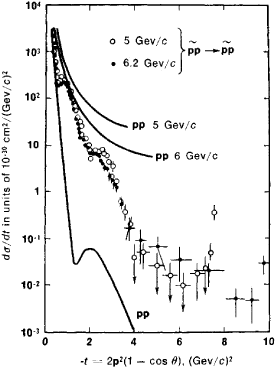Figure 3. Differential cross sections at various energies E for the scattering of protons (p) and antiprotons (p̃) by protons; the cross sections are plotted as a function of the square of the transferred momentum: —t = 2p2 (1 — cos Θ), where p is the momentum and β is the scattering angle in the particles’ center-of-mass system. The angular dependence of the cross section is the same as for diffraction by a black sphere with absorptivity smoothly decreasing toward the edges—that is, a sphere with blurred edges.

The experimental study of hadron elastic scattering processes has confirmed that such scattering, in its general features, exhibits a diffraction character. In some cases, secondary diffraction maxima can even be observed (Figure 3).

As the energy is increased, however, more complicated regularities are observed that suggest the existence of interaction mechanisms with different ranges, which depend on the interaction energy.

Isotopic-spin invariance. The first internal symmetry of strong interactions to be experimentally detected was the charge independence of the nuclear forces. According to this concept, nuclear interactions of protons with protons, of neutrons with neutrons, and of neutrons with protons in identical states are the same—that is, are independent of the electric charge of the nucleons.

The charge independence of the nuclear forces is a manifestation of a more general symmetry of strong interactions called isotopic-spin, or isospin, invariance. Isotopic-spin invariance means that the strong interaction between nucleons does not change if, instead of the wave functions of the proton (p) and neutron (n), we take a superposition of their states (p′) and (n′):

(1) P′ = αp + βn

n′ = γp + δn

where α, β, λ, and δ are certain complex numbers (here, the wave functions of the particles are designated by the symbols of the corresponding particles). Such a transformation obviously is more general in character than the simple replacement of protons by neutrons or of neutrons by protons. The total probability for a nucleon to be in the state of a proton or neutron under this transformation should not vary—that is, ǀp′ǀ2 + ǀn’ǀ2 = ǀpǀ2+ ǀnǀ2. It follows that the matrix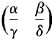of the transformation must be unitary. Furthermore, the law of conservation of baryonic charge is related to the invariance of an interaction with respect to the multiplication of the wave functions of the neutron and proton by an identical phase factor eix, where ξ is an arbitrary number. Thus, this factor can be eliminated from transformation (1), and the determinant of the transformation matrix can be set equal to 1.

The group of transformations carried out by means of second-order unitary matrices whose determinants are equal to 1 is called the SU(2) group. It is mathematically equivalent to the group of rotations in the abstract three-dimensional space known as isotopic, or isospin, space. The symbol U(2) indicates that unitary second-order matrices are involved here, and the symbol S designates the special case of the transformation where the determinant of the matrix is equal to 1. The group SU(2) can be characterized by three independent parameters, such as the angles of rotation with respect to the three axes of isotopic space. In order that the interaction forces between nucleons do not vary under transformation (1), the following two conditions must be fufilled: first, besides charged pions (π±), neutral pions (77°) of the same mass must participate in the transfer of nuclear forces; second, the interactions of nucleons with pions must be invariant with respect to rotation in isotopic space. The existence of the π0meson, which was discovered after the charged pions, was theoretically predicted on the basis of this conclusion. In addition, the conclusion provided grounds for indicating the relation between the probabilities of different processes involving pions and nucleons. The experimental study of such processes has confirmed to a high degree of accuracy the invariance of strong interactions for pions and nucleons.

After the discovery of strange particles (K-mesons and hy-perons) and the establishment of the strangeness quantum number specific to hadrons, it was experimentally proved that the isotopic-spin invariance of strong interactions also holds for strange particles. Like pions and nucleons, strange particles, as well as the resonances discovered later, form groups of particles having the same quantum numbers and approximately equal masses. These groups are called isotopic-spin multiplets. The slight differences in mass of the particles making up a multiplet can be attributed to the electromagnetic interaction. The electric charges Q of the particles forming a single multiplet are determined by a formula established by M. Gell-Mann and K. Nishijima: Q = 1/2 (B + S) + I3. Here, B is the baryon number, and 5 is the strangeness; B and S are the same for all the particles in a multiplet. The quantity I3 can assume, at intervals of 1, all values from some maximum, integral or half-integral value I to the minimum value - I—that is, I3 = I, I - 1, …, -I, for a total of 2I + 1 values. The quantity I is called the isotopic spin and is an important characteristic of hadrons. It determines the number of particles in an isotopic-spin multiplet. If the particles forming an isotopic-spin multiplet are regarded as different charge states of the same particle, then I determines the number of charge states of the particle. The quantity B + S = Y is called the hypercharge. It determines the average electric charge <Q> of the isotopic-spin multiplet: <Q> = Y/2. This average charge is the algebraic sum of the electric charges of the particles divided by the number of particles in the multiplet.

The unitary symmetry SU(3). The discovery of a large number of resonances and the determination of the resonances’ quantum numbers showed that the hadrons forming different isotopic-spin multiplets can be combined into broader groups of particles having the same spin, parity, and baryon number but with different hypercharges. Such groups are called supermultiplets. For example, what is known as the baryon octet is a supermultiplet consisting of the eight baryons with spin 1/2 and even parity: the nucleons N (proton, neutron), with isotopic spin I = 1/2 and hypercharge Y = 1; the Σ-hyperons (Σ+, Σ0, Σ-), with I = 1 and Y = 0; the Λ-hyperon, with I = 0 and Y = 0; and the Ξ-hyperons (Ξ0, Ξ-), with I = 1/2 and Y = - 1. The baryons with spin 3/2 and even parity form a decuplet. This supermultiplet includes the resonances Δ (Δ+ +, Δ+, Δ0, Δ-), with I = 3/2 and Y = 1; the resonances Σ* (Σ + *, Σ0*, Σ-*), with I = 1 and I = 0; the resonances Ξ* (Ξ0*, Ξ-*), with I = 1/2 and Y = - 1; and the Ω--hyperon, with I = 0 and Y = - 2. The mesons form supermultiplets in an analogous manner. For example, the eight mesons with zero spin and odd parity form a meson octet: the π-mesons (π+, π°, π), with I = 1 and Y = 0; the K-mesons (K+, K0, K-, K̃0), with I = 1/2 and Y = ± 1; and the η-meson, with I = 0 and Y = 0.

Since, however, the masses of the particles making up a single supermultiplet differ appreciably, the symmetry of the strong interaction, as a result of which the groups of similar particles exist, is clearly an approximate rather than an exact symmetry. The strong interaction can be regarded as being made up of a superstrong interaction, which has a high degree of symmetry, and a moderately strong interaction, which violates symmetry. The superstrong interaction is independent of both the electric charge and the hypercharge of the particles involved. If only the superstrong interaction existed, the masses of all the particles in a supermultiplet would be the same. The observed differences in the masses of particles with different hypercharges is due to the existence of the moderately strong interaction, which depends in a definite way on the hypercharge and isotopic spin. The composition of the experimentally detected supermultiplets—that is, the number of particles and the quantum numbers of the particles—can be explained if the superstrong interaction is assumed to be invariant with respect to transformations of the SU(3) group, which include the isotopic-spin transformations of SU(2) as a subgroup.

To explain the experimentally observed SU(3) symmetry of strong interactions, the quark model has been advanced. According to this hypothesis, hadrons consist of three types of fundamental particles—the quarks p, n, and λ. Moreover, as was the case for transformation (1), the strong interaction does not change when the wave function of each of these particles is replaced by a superposition of the wave functions of the remaining particles. Since in the present case the transformation is accomplished by means of third-order unitary matrices of determinant 1, the invariance of the strong interaction with respect to this transformation signifies the existence of SU(3) symmetry. In actuality, particles in the same multiplet that have different hypercharges and isotopic spins exhibit different masses. This observed violation of SU(3) symmetry can be explained in a satisfactory manner by assuming that the mass of the strange λ-quark is greater than that of the p- and n-quarks.

The quark hypothesis was advanced to explain the observed composition of hadron supermultiplets, but it also makes possible an explanation of a number of dynamic regularities of strong interactions.

Various generalizations of the original quark hypothesis exist. It has also been argued that quarks can exist only in bound states and cannot be observed as free particles.

Since perturbation theory, which is so effective in quantum electrodynamics, cannot be used to describe strong-interaction processes, the main directions of development in the present-day theory of strong interactions involve the use of the general principles of quantum field theory, the symmetry of the strong interaction, and various model representations that to varying degrees allow for the multiparticle character of the interaction.

The processes occurring during the interaction of particles can be described in the most general form by means of the scattering matrix, or S-matrix, which expresses the state of a system before a reaction in terms of the state after the reaction. The importance of the scattering matrix in relativistic quantum mechanics was suggested by W. Heisenberg in 1943. The elements of the scattering matrix are the probability amplitudes for transitions from different initial states to different final states of the system. Thus, specification of the scattering matrix completely determines the probabilities of various reaction channels in the interaction of particles.

The general principles of quantum field theory make possible the derivation of equations connecting the characteristics of various strong-interaction processes and permit the establishment of certain restrictions on the character of strong-interaction processes at high energies. These equations are the basis for the construction of various approximate models that describe experimentally the observed regularities in strong-interaction processes.

One of the basic principles of quantum field theory is that the scattering matrix is unitary. In other words, the sum of the probabilities of all possible transitions that can occur in a system must be equal to unity. It is, of course, assumed that the set of possible states of the system is complete. An important consequence of the unitarity condition is the optical theorem, according to which the total effective scattering cross section of particles depends on the imaginary part of the elastic scattering amplitude in the forward direction. The unitary condition also limits the magnitude of the cross section for individual partial waves, that is, waves with a definite orbital angular momentum (seeSCATTERING OF MICROPARTICLES).

Furthermore, the fulfillment of the laws of the special theory of relativity (relativistic invariance, or Lorentz invariance) provides the possibility of formulating a microcausality principle for elementary processes of strong interactions (seeMICROCAUSALITY CONDITION). According to the special theory of relativity, two events separated by a spacelike interval cannot be causally related, since the distance between the events is greater in this case than the path that any signal can traverse during the time interval between the events. If, however, events are separated by a timelike interval, then only events preceding a given event in time can be its cause. This general form of the microcausality principle imposes certain restrictions on the analytic structure of the functions describing causally related events. This fact was noted already in the classical electrodynamics of continuous media in describing the dependence of the dielectric constant (and consequently the refractive index) of a substance on the frequency ω of the electromagnetic field. The dependence (ω) is known as the dispersion of the medium. For variable fields, the value of the electric flux density D(t) at some moment t is determined by the values of the electric field strength E at preceding moments t′ (according to the causality principle, t′ ≤ t). The general linear relation between these quantities can therefore be written as follows: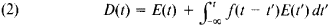In this expression, f(tt′) is a function determined by the internal structure of the dielectric. It is not necessary to know the specific form of this function to make further conclusions. What is important is that the function f(t - t′) depends only on the time difference (t - t′). This fact follows from translational invariance with respect to time—that is, from the independence of the selection of the origin of the time axis. In accordance with the causality principle, integration with respect to t′ is carried out up to the time t.

For the Fourier components D(ω) and E(ω) of D(t) and E(t), the following equation holds:

(3) D(ω) = ∊(ω)E(ω)

Here, the dielectric constant ∊(ω) is the complex function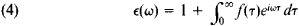The limits of integration τ ≥ 0 follow from the causality condition. Equation (4), which is defined for real values of ω, can be extended into the domain of complex values of the variable ω. If we set ω = ω′ + iω″, where ω′ and ω″ are real numbers determining, respectively, the real and imaginary parts of ω, then the factor e-ω″τ appears in the integral of equation (4); this factor ensures convergence of the integral when ω″ > 0 and e-ω″τ < 1. It thus follows from the causality principle that the function (ω) is analytic in the upper half plane of the complex variable ω (ω″ > 0). This transition to the nonphysical domain of complex values of ω has profound significance, since the Cauchy theorem, which permits the value of a function for any value of the variable to be expressed in terms of the Cauchy integral of the function, is valid for analytic functions. By selecting a real value of the variable, it is possible to obtain equations for the physical quantities actually measured. The dispersion relations were obtained in this manner. They permit, for example, the real part (Re) of the dielectric constant to be expressed in terms of an integral of its imaginary part (Im):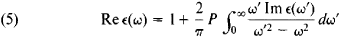where the symbol P denotes the principal value of the integral, that is, the value excluding the singularity ω′ = ω. It is important that the real and imaginary parts of ∊(ω) can be directly measured experimentally [Im ∊(ω) is connected with the absorption of electromagnetic waves.]

The determination of the analytic properties of the particle scattering amplitude is a much more complicated problem. Fundamental work in this regard was done by N. N. Bogoliu-bov on the basis of the microcausality principle that he formulated for the S-matrix method. Let us consider an elastic scattering reaction that causes two particles a and b with the initial four-momenta pa and pb, respectively, to move to a state with the four-momenta p′a and p′b. The four-momentum of a particle includes the particle’s energy E and its three-momentum p. The square p2 of the four-momentum, in units such that the speed of light c = 1, is defined as p2 = E2 - p2 and is equal to the square of the mass of the particle: p2 = M2. The law of conservation of energy and momentum in a scattering reaction can be written in the form of the equality pa + pb = p′a + p′b. The elastic scattering of particles can be described most simply in the center-of-mass system of the colliding particles. In this system, pa + Pb = Pa + Pb = 0. In other words, the momenta of the particles after the collision are directed in opposite directions and are equal in absolute value to the initial momenta: ǀpaǀ = ǀpbǀ = ǀpaǀ = ǀp′bǀ (see Figure 2). The scattering amplitude is a function of two variables: the energy of the system E and the angle θ at which one of the particles is deflected as a result of scattering. These variables can be expressed in terms of two independent relativistically invariant quantities: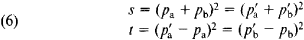In the center-of-mass system, the quantity s is equal to the square of the total energy of the system: s = (Ea + Eb)2. The quantity t in such a system is equal to the negative of the square of the transferred three-momentum: t = - (p′a - pa)2. In terms of the scattering angle Θ, t = - 2p2(1 - cos Θ), where ρ is the momentum of the particles in the center-of-mass system.

In addition to the quantities s and t, a third relativistically invariant quantity u is introduced:Because of the law of conservation of energy-momentum, the relation between u, s, and t is given by the equation s + t + u = 2ma + 2mb, where ma and mb are the masses of a and b. In processes of the elastic scattering of particles, the range of the quantity s is limited by the inequality s ≥ (ma + mb)2, and the range of the t is limited by the inequalities 0 > t > - 4p2. These ranges of the variables are called the physical regions. The scattering amplitude for a fixed transfer of momentum t can be extended into the complex region with respect to the energy variable s and turns out to be connected with the scattering amplitude of the antiparticles. To explain this connection, let us consider two examples of scattering reactions. The first is the elastic scatterinp of π+-mesons by protons:

(I) π+(p) + p(q) → π+(p′) + p(q′)

the quantities in parentheses are the four-momenta of the particles). The second is the reaction

(II) π-(− p) + p(q) → π-(− p′) + p(q′)

which is obtained from (I) by replacing the meson (π+) with its antiparticle (π-) and by changing the signs of the meson’s four-momenta: p → − p, p′ → −p′. When we move from process (I) to process (II), the variable t remains unchanged, and s and u change places. The physical regions of the two processes correspond to two different, nonoverlapping ranges of the kinematic variables s and u.

Bogoliubov’s proof of the analyticity of the amplitude in the complex plane of the variable s permits the assertion that the amplitudes of processes (I) and (II) are the limiting values of the single analytic function F,(s) in different regions of the variable s with branch cuts on the real axis (Figure 4). The right-hand branch cut is determined by the condition s ≥ (M + μ)2, where M and μ are the masses of the proton and pion. The left-hand branch cut is determined by the condition u = 2M2 + 2μ2 - s - t ≥ (M + μ)2. On the upper side of the right-hand branch cut, F,(s) coincides with the amplitude T(s, t) of process (I):

Ft(s + i0) = T(I) (s, t)

On the lower side of the left-hand branch cut, F,(s) coincides with the amplitude of process (II):

Ft(u + i0) = T(II) (s, t)

From these considerations there follows the equation for crossing symmetry:

TII(s, t) = T(I)* (u, t)

This equation gives the relation between the value of the amplitude of one process in its physical region and the value of the amplitude of the other process outside the other process’s physical region. The crossing symmetry equation therefore would have no meaning if there did not exist a continuation of the amplitude of process (I) from its physical region into the left-hand branch cut.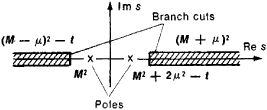Figure 4

Of great importance for the determination of the singularities of the analytic function Ft(s) is the extension of the unitarity condition of the S-matrix into the nonphysical region of kinematic variables, which lies outside the physical regions defined by the laws of conservation of energy and momentum for the initial and final states. Suppose, for example, that the two particles a and b can become the virtual particle c as a result of a strong interaction: a + b → c. It follows from the unitarity condition that the amplitude of the scattering process a + b → a + b has a pole with respect to the variable s at the value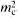, where mc is the mass of particle c. When mc < ma + mb, this pole lies in the nonphysical region of the elastic scattering process a + b → a + b; as noted above, the physical region begins at s = (ma + mb)2. If, however, mc > ma + mb, particle c is unstable with respect to the decay (through the strong interaction) c —> a + b—that is, c is a resonance, and the pole of the amplitude lies on the Riemann surface’s nonphysical sheet corresponding to an analytic continuation of the amplitude through the branch cut in the complex s-plane (seeANALYTIC FUNCTIONS).

As has been noted, the amplitude singularities connected with the formation of virtual particles lie in the nonphysical region. This fact has a simple meaning. The production of virtual particles is accompanied by a violation of the law of conservation of energy; this violation occurs for a short period in accordance with the uncertainty principle. Since physical regions are determined by the laws of conservation of energy-momentum and by the condition of stability of the initial and final particles in strong-interaction processes, the formation of virtual states is associated with kinematic-variable values that lie outside these regions. Thus, it is the nonphysical regions of the kinematic variables that contain information on the virtual-particle exchange processes by means of which the strong interaction is carried out.

In addition to the poles, the scattering amplitude may have other singularities. Consider, for example, an inelastic process, such as a + b → c + d. At an energy corresponding to the threshold of the process—that is, when s = (mc + md)2—the amplitude of the reaction a + b → a + b has a branch point. When (mc + md) > (ma + mb), these singularities lie in the physical region of the process a + b → a + b and lead to irregularities in the behavior of the effective scattering cross section of the particles a + b near the production threshold of the particles c and d. These irregularities are due to the appearance of a new reaction channel.

By assuming that the scattering amplitude as a function of the variables s, t, and u has only those singularities that arise from the generalized condition for unitarity of the S-matrix, we arrive at the conclusion that a single analytic function f(s, u, t) in different regions of the variables describes the three different processes

(I) a + b→c + d

(II) c + b→ā + d

(III) a + b→ã + c

(a tilde over the symbol for a particle indicates the antiparticle), as well as the reverse reactions. This hypothesis—in contrast to, for example, the coupling of the scattering channels π+ + p → π+ + p and π- + P → π p for fixed transferred momenta—has not been rigorously substantiated on the basis of the principles of quantum field theory. The hypothesis’s validity has been confirmed only on the basis of a consideration of lower orders of perturbation theory. Nonetheless, the hypothesis is often used as a postulate of present-day theory.

The assumption that a single analytic function in different regions of its variables corresponds to the amplitudes of the physical processes (I), (II), and (III) permits dispersion relations to be written for the function with respect to two complex variables: (s, t), (s, u), (t, u). By means of this representation, which is known as the Mandelstam double spectral representation, an analytic continuation of the amplitude can be carried out in the regions of the variables s, t, u that correspond to the nonphysical regions of reactions (I), (II), and (III). This representation thereby becomes the basis for a dynamic description of strong interactions that does not employ perturbation theory. Indeed, as noted above, amplitude singularities lying in nonphysical regions correspond to the exchange of virtual particles, through which the strong interaction is carried out. Thus, the nonphysical region of one reaction channel can have a considerable effect on the behavior of the amplitude in the physical region of another channel.

Some results following from the analytic properties of the scattering amplitude were rigorously obtained on the basis of quantum field theory. The analyticity of the amplitude with respect to energy permits the construction of dispersion relations, by means of which the real part of the zero-angle scattering amplitude is expressed in terms of an integral of the imaginary part of the amplitude. According to the optical theorem, the imaginary part of the amplitude of forward elastic scattering in the physical region (in the right-hand branch cut of the complex s-plane) is connected with the total scattering cross section of the particle; in the left-hand branch cut, because of crossing symmetry, the imaginary part is expressed in terms of the total scattering cross section of the antiparticle. The real part of the amplitude can thus be represented in the form of a dispersion integral that includes the difference in the cross sections for the particles and antiparticles on the same target. Moreover, the dispersion relation includes the contribution from poles lying in the nonphysical region—for example, in the case of pion-nucleon scattering, the contribution from the pole corresponding to the virtual transformation π + N→N→π + N. An important consequence of the dispersion relations is the possibility of determining from experimental data the nucleon-pion coupling constant and of verifying its universality in various reactions. Another consequence pertains to the asymptotic behavior of the total scattering cross sections of particles and antiparticles at high energies. I. Ia. Pomeranchuk proved, on the basis of the dispersion relations, an important theorem by assuming that the elastic scattering of high-energy hadrons has the character of diffraction scattering with a constant range and that the total cross sections tend toward constant limits as the energy increases. The theorem states that these limits are equal for the total cross sections of particles and antiparticles for scattering by the same target—for example, σ(π+ + p)→ σ(π- + p).

It has been shown on the basis of the principles of quantum field theory that the scattering amplitude is an analytic function of the variable z = cos θ within an ellipse whose semimajor axis extends into the nonphysical region z > 1 and is determined by the smallest mass of the particles existing in the t-channel of the reaction, that is, the particles carrying the strong interaction. Since the amplitude in the ellipse is an analytic function, the partial scattering amplitudes corresponding to the collision of particles with relative orbital momentum l decrease exponentially for large l, beginning at a value proportional to(ln s)/2μ, where μ is the smallest mass of the particles carrying the interaction. This result corresponds to qualitative considerations requiring the range of an interaction caused by the exchange of some particles to be inversely proportional to the mass of the particles carrying the interaction. Suppose the interaction has the range R0. When particles with momentum p collide, the maximum orbital moment l0 at which the interaction still occurs is determined by the relation ǀpǀR0ℏl0—that is, R0 ~ (In s)/μ. Thus, the analytic properties of the scattering amplitude as a function of the transferred momentum permit establishment of the maximum range of the interaction. The maximum range, however, may increase in proportion to In s as the energy increases. The total interaction cross section, therefore, cannot increase with increasing energy more rapidly than ln2s, and the diffraction cone in elastic scattering cannot narrow faster than I n2s. A number of theorems follow from the analytic properties of the scattering amplitude and the short-range character of strong interactions. For example, the differential cross sections for the scattering of particles and antiparticles by the same target are equal. Another example is the generalization of the Pomeranchuk theorem to the case of interaction cross sections and ranges that increase with energy.

On the basis of the dispersion relations and the unitary condition, a theory has been developed that describes a number of processes in the energy range up to approximately 1 Gev. These processes include the production of π-mesons by gamma quanta (photoproduction) and the scattering of π-mesons by nucleons and π-mesons.

The scattering amplitude of a particle is expressed in terms of the partial amplitudes fl(E) corresponding to the different orbital momenta l of the collision. In accordance with their quantum-mechanical meaning, the quantities l can assume only positive integral values. For the case, however, of the scattering of a particle by some spherically symmetric potential, partial amplitudes can formally continue into the region of complex values of l. In addition the partial amplitude can be shown to be an analytic function of l in the right half plane of the complex variable l (more accurately, when Re l > - 1/2). The method of analytic continuation with respect to l was introduced by the Italian physicist T. Regge. In the case of short-range potentials, including the Yukawa potential V = ge-μr/r and the superposition of such potentials, he showed that only the poles li = li(E) whose position in the complex plane is energy-dependent can be singularities of the partial amplitude to the right of the line Re l = − 1/2. These poles are called Regge poles and have a simple physical meaning: stable bound states and resonances are obtained directly from Regge poles. Suppose the quantity li(En) is equal to a positive integer l at certain energy values E = E below the threshold—that is, when E < 0 for the scattering of a particle by an external field that vanishes at infinity or when E < ma + mb for processes of the collision of particles a and b. The system then has stable bound states with the orbital momentum l. If, at energy values E = Er above the threshold, Re li (Er) is equal to a positive integer, then the system has resonances. The function li(E) is called the Regge trajectory. It should be noted that above the reaction threshold this function is complex. If the exchange interaction is allowed for, a situation results in which one Regge trajectory exists for bound states and resonances with even orbital momenta and another exists for odd momenta.

To illustrate the concept of the Regge trajectory, let us consider the scattering of an electron in the Coulomb field of the nucleus of a hydrogen-like atom. The energy levels in this case are given by Bohr’s formula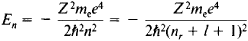where n is the principal quantum number and Z is the atomic number (seeATOM). Bohr’s formula yields the equationin which to positive integral values of l there correspond definite energy levels En of the system.

For values of E > 0 (above the threshold), we have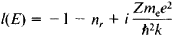where k is the wave number, related to the energy by the equation E = 2k2/2me. When E > 0, Re l(E) is not equal to a positive integer. This fact means that the system does not have resonance states.

Regge trajectories have been used to classify stable nuclear particles and resonances. In contrast to the classification based on particle symmetry, this classification rests on interaction dynamics. By using the Regge trajectory α(E), it is possible to systematize particles having the same intrinsic characteristics but differing by an even number of spin values. Groups of particles forming supermultiplets therefore should recur with different values of spins, which differ by an even number. In other words, in addition to the baryon octet with spin 1/2, there should exist baryon octets with spin 5/2, 9/2, and so on. A sort of analogue to Mendeleev’s periodic table is thus obtained. The Regge trajectories uniting particles with the same intrinsic characteristics are analogous to the columns of the periodic table.

Experiment has shown that the Regge trajectories for particles are approximately linear functions of the squares of the particles’ masses (Figure 5). The trajectory on which resonances with quantum numbers (other than l) of a vacuum (I = J = 0, parity P = + 1) lie plays an important role in the phe-nomenological description of scattering processes. Called theFigure 5. Regge trajectories for Δ-resonances

vacuum, or Pomeranchuk, trajectory, it determines the total cross section at very high energies. The processes in which exchange of charge, strangeness, and other quantum numbers occurs (such as π+ p → π0 + n) are described in phenomenolog-ical analysis by reggeons, which are Regge trajectories with the corresponding quantum numbers.

In relativistic theory, branch points appear in addition to Regge poles. The structure of singularities in the complex l-plane, however, has not yet been completely elucidated.

Various reggeon models for the description of scattering and multiple production processes at high energies have been constructed on the basis of hypotheses concerning the character of the singularities of partial amplitudes.

Strong-interaction processes have also been successfully studied through the use of the multiperipheral model and the description of reactions by means of quasi potentials, which allow for the absorption of particles.

Sum rules that integrally connect resonances in one reaction channel with resonances in a cross channel have been constructed on the basis of the dispersion relations and an assumption on the character of the singularities in the l-plane. This approach is based on the concept of global duality. A further development of this approach is the hypothesis of local duality, according to which the amplitude of the process in each reaction channel is determined at low energies by the resonances existing in this channel and at high energies by the resonances from cross channels. The duality hypothesis is the starting point for the construction of various duality models.

In several extremely productive areas of investigation of strong interactions, the internal symmetries of such interactions are used to provide a dynamic description of the processes involved. In particular, in current algebra steps have been taken toward combining group theoretical methods for studying symmetries and the field theory concepts used in the dispersion relation method. The principal idea underlying current algebra is the existence of conserved hadron currents. One such current is the electromagnetic, or vector, current, whose law of conservation corresponds to that of the electric charge. Because of the isotopic-spin invariance of strong interactions, the hypothesis has been advanced that the charged vector current is also conserved. This current is the isotopic partner of the electromagnetic current and corresponds, for example, to neutron-to-proton transformations and the reverse transformations. The conservation of such a charged vector current has been well verified in weak interactions of hadrons with leptons. By taking into account the SU(3) symmetry of the strong interaction, it is possible to hypothesize the conservation of some other vector currents—in particular, those corresponding to transformations of nucleons to hyperons. In addition to vector currents, there exist axial vector hadron currents. For example, the charged axial vector current corresponding to the neutron-proton transformation, together with the charged vector current, determines the weak interactions of nucleons. Strictly speaking, the axial vector hadron current does not appear to be conserved. Experimental data, however, support the assumption that the violation is minimal and vanishes under conditions where the mass of the pion may be ignored. The theory of the partially conserved axial vector current, a number of consequences of which are in good accord with experimental data, is based on this assumption. The SU(3) symmetry of strong interactions can be used to establish relations, called commutation relations, between the operators corresponding to vector and axial vector currents. Current algebra is based on these relations. A rigorous substantiation of the commutation relations has not yet been provided; the quark hypothesis, for example, has been used in support of them. Nonetheless, their use based on field theoretical methods has led to a number of important predictions that have been experimentally verified. The application of current algebra to processes of weak and electromagnetic interactions of leptons with hadrons has been especially productive.

The theory of gauge, or compensating, fields is another important area of investigation of strong interactions. According to this theory, to quantities conserved in the strong interaction—such as baryon number, electric charge, isotopic spin, and hypercharge—there corresponds an interaction carried by particles whose spin is equal to 1 (vector mesons). Electromagnetic interactions are known to be carried by photons, which have the spin 1. In addition, substantial grounds exist for assuming that weak interactions are carried by the vector particles known as intermediate vector bosons. Thus, the successful development of gauge theories of strong interactions has made possible the hypothesis that a profound internal relation exists between the three types of interactions and has raised hopes for the creation of a unified theory of the interactions.

### REFERENCES

Bogoliubov, N. N., B. V. Medvedev, and M. K. Polivanov. Voprosy teorii dispersionnykh sootnoshenii. Moscow, 1958.
Logunov, A. A., and Nguyen Van Hieu. “Osnovnye tendentsii ν razvitii teorii sil’nykh vzaimodeistvii.” Fizika ekmentarnykh chastils i atomnogo iadra (EChAIa), 1974, vol. 5, issue 3.
Logunov, A. A., M. A. Mestvirishvili, and O. A. Khrustalev. “Ogranicheniia na povedenie sechenii uprugikh i neuprugikh protsessov.” Fizika elementarnykh chaslits i atomnogo iadra (EChAIa), 1972, vol. 3, issue 1.
Teoriia sil’nykh vzaimodeistvii pri bol’shikh energiiakh: Sb. statei. Moscow, 1963. (Translated from English.)
Schweber, S., H. Bethe, and F. de Hoffmann. Mezony ipolia. Moscow, 1957. (Translated from German.)
Collins, P. and E. J. Squires. Poliusa Redzhe ν fizike chaslits. Moscow, 1971. (Translated from English.)
Feynman, R. Vzaimodeistvie fotonov s adronami. Moscow, 1975. (Translated from English.)
Eden, R. Soudareniia elementarnykh chastits pri vysokikh energiiakh. Moscow, 1970. (Translated from English.)

A. A. LOGUNOV and S. S. GERSHTEIN

References in periodicals archive ?
Rockenbauer felt that the aforementioned problems added more fuel to the usefulness of a study especially when no weak and strong interactions are considered for the neutrinos and quarks.
However, a strong interaction exists among the process input variables.
Due to the strong interaction of [Fe.sup.2+] with these atoms of the membrane in the hydrate, it is very difficult for these hydrates to get rid of the interaction with their surroundings and to diffuse in the membrane.
The level of the chemical also showed a strong interaction with the high-risk apolipoprotein E (apo E) epsilon-4 allele in influencing Mini-Mental State Examination (MMSE) scores, wrote Dr.
However, researchers identified a strong interaction of diabetes mellitus (p = 0.0134) on the relationship of adherence to MeD with TO; high adherence to MeD was associated with a lower likelihood of ICI in non-diabetic participants (OR 0.81; 95% CI 0.70-0.94; p = 0.0066) but not in diabetic individuals (OR 1.27; 95% CI 0.95-1.71; p = 0.1063).
The excess viscosities, excess square of refractive index and excess activation energy are all positive indicating strong interaction between the alcohols and amines molecules.
It provides our best understanding of the strong interaction, describing the hadrons (such as the particles that compose the atomic nucleus, the protons and neutrons) in terms of the elementary quarks and gluons and their interactions.
THERE'S a chance that exiting Typhoon "Igme" (international name Tembin) will meet the approaching Typhoon "Julian" (Bolaven) in the next 24 hours but weathermen do not see strong interaction between them.
It allows people to express their opinion and it creates strong interaction between government and the public," said Xie Yungeng, an expert in public opinion and new media from Shanghai Jiaotong University ( ANI )
Sodini said the objective of MEDRC is to provide precom-petitive medical electronic device research involving strong interaction among medical device and microelectronics companies as well as physicians and clinicians.
"With its back-to-the-basics approach and strong interaction with the Fleet, CSS has become a pillar of excellence, ensuring our MCs are trained and ready to perform the mission."
Maliki concluded his speech saying: "the strong interaction between the government and the Parliament can't be ignored, in order to achieve the ambitions and hopes of our people, who have suffered a lot and necessitate us to compensate what they had suffered from loss of public services, violation of human rights, freedoms and sacred demands, during 35 years of the dark Baath era."

Site: Follow: Share:
Open / Close